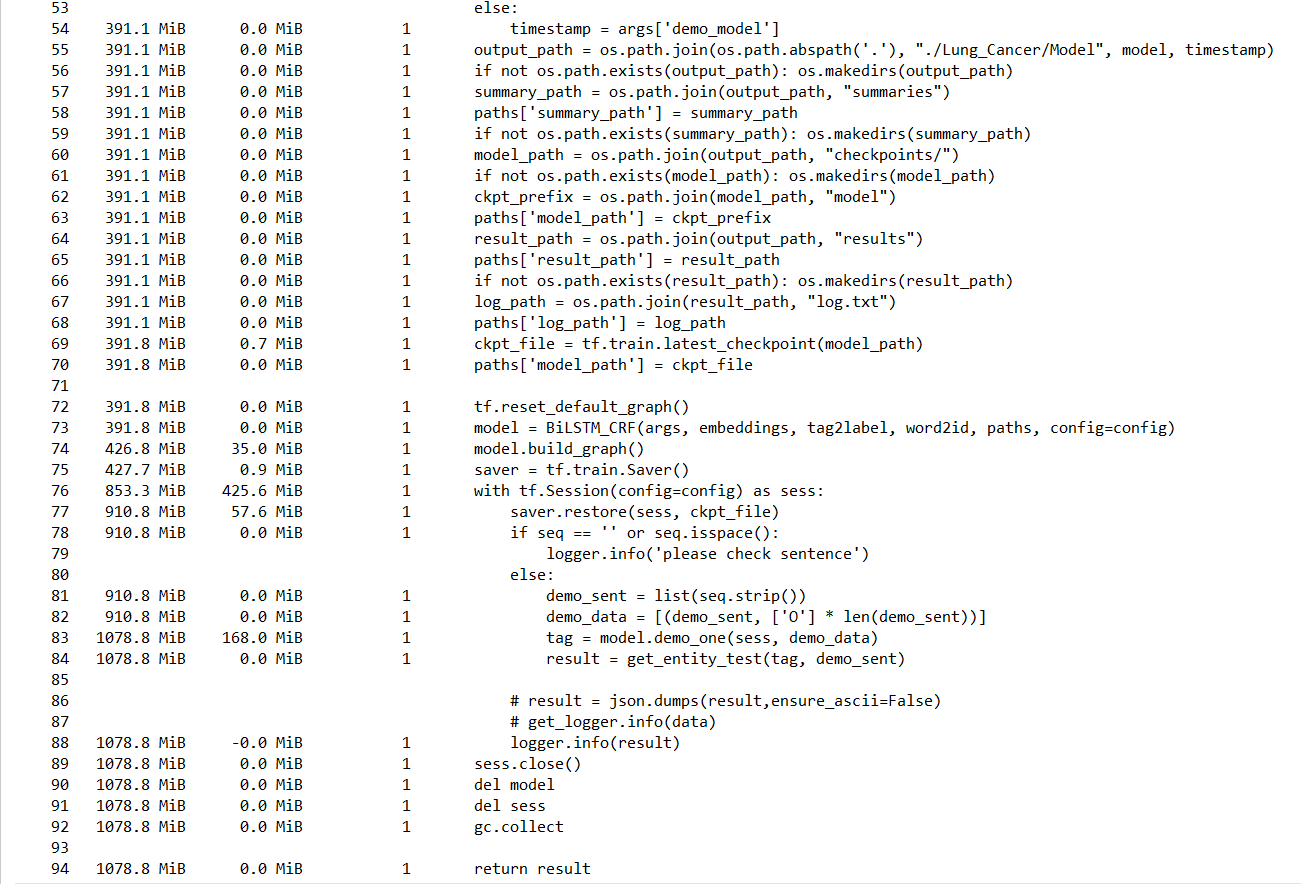2021-03-04 16:17

# Tensorflow Session关闭后内存不释放• 点赞
• 写回答
• 关注问题
• 收藏
• 复制链接分享
• 邀请回答

#### 1条回答

• ``````import tensorflow as tf
import multiprocessing
import numpy as np

def run_tensorflow():

n_input = 10000
n_classes = 1000

# Create model
def multilayer_perceptron(x, weight):
# Hidden layer with RELU activation
layer_1 = tf.matmul(x, weight)
return layer_1

# Store layers weight & bias
weights = tf.Variable(tf.random_normal([n_input, n_classes]))

x = tf.placeholder("float", [None, n_input])
y = tf.placeholder("float", [None, n_classes])
pred = multilayer_perceptron(x, weights)

cost = tf.reduce_mean(tf.nn.softmax_cross_entropy_with_logits(logits=pred, labels=y))
optimizer = tf.train.AdamOptimizer(learning_rate=0.001).minimize(cost)

init = tf.global_variables_initializer()

with tf.Session() as sess:
sess.run(init)

for i in range(100):
batch_x = np.random.rand(10, 10000)
batch_y = np.random.rand(10, 1000)
sess.run([optimizer, cost], feed_dict={x: batch_x, y: batch_y})

print "finished doing stuff with tensorflow!"

if __name__ == "__main__":

# option 1: execute code with extra process
p = multiprocessing.Process(target=run_tensorflow)
p.start()
p.join()

# wait until user presses enter key
raw_input()

# option 2: just execute the function
run_tensorflow()

# wait until user presses enter key
raw_input()``````

这样使用session会解决你的问题

https://github.com/tensorflow/tensorflow/issues/1727

这里是当时的issue

点赞 1 评论 复制链接分享